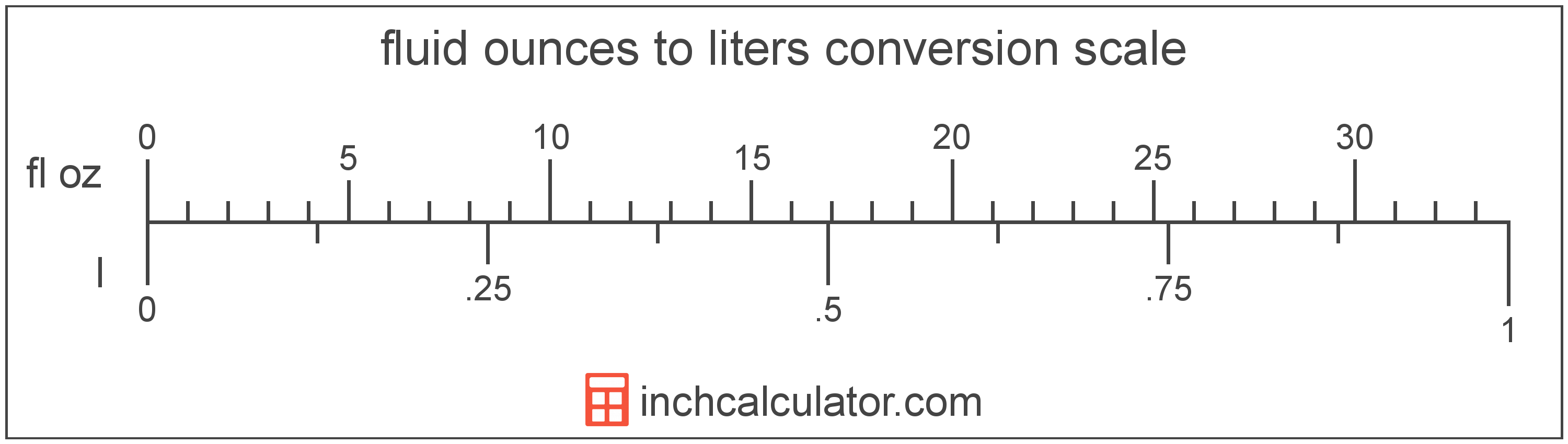# Liters to Fluid Ounces Converter

Enter the volume in liters below to get the value converted to fluid ounces.

Results in Fluid Ounces:1 L = 33.814023 fl oz

Do you want to convert fluid ounces to liters?

## How to Convert Liters to Fluid Ounces

To convert a measurement in liters to a measurement in fluid ounces, multiply the volume by the following conversion ratio: 33.814023 fluid ounces/liter.

Since one liter is equal to 33.814023 fluid ounces, you can use this simple formula to convert:

fluid ounces = liters × 33.814023

The volume in fluid ounces is equal to the volume in liters multiplied by 33.814023.

For example, here's how to convert 5 liters to fluid ounces using the formula above.
fluid ounces = (5 L × 33.814023) = 169.070114 fl oz### How Many Fluid Ounces Are in a Liter?

There are 33.814023 fluid ounces in a liter, which is why we use this value in the formula above.

1 L = 33.814023 fl oz

## What Is a Liter?

A liter is a unit of volume equal to 1,000 cubic centimeters or 0.264172 US gallons. The liter is a special name defined for the cubic decimeter and is exactly equal to the volume of one cubic decimeter (1 decimeter is 1/10 of a meter, or 10 centimeters).

The liter is an SI accepted unit for volume for use with the metric system. A liter is sometimes also referred to as a litre. Liters can be abbreviated as L, and are also sometimes abbreviated as l or . For example, 1 liter can be written as 1 L, 1 l, or 1 ℓ.

## What Is a Fluid Ounce?

The US fluid ounce is a unit of volume equal to 1/16 of a pint or 1/8 of a cup. The fluid ounce is sometimes referred to as just an "ounce" but should not be confused with the unit of weight. One fluid ounce is equal to approximately 29.5735 milliliters, but in nutrition labeling, one fluid ounce is rounded to exactly 30 milliliters.

The fluid ounce is a US customary unit of volume. Fluid ounces can be abbreviated as fl oz, and are also sometimes abbreviated as oz fl. For example, 1 fluid ounce can be written as 1 fl oz or 1 oz fl.

## Liter to Fluid Ounce Conversion Table

Table showing various liter measurements converted to fluid ounces.
Liters Fluid Ounces
1 L 33.81 fl oz
2 L 67.63 fl oz
3 L 101.44 fl oz
4 L 135.26 fl oz
5 L 169.07 fl oz
6 L 202.88 fl oz
7 L 236.7 fl oz
8 L 270.51 fl oz
9 L 304.33 fl oz
10 L 338.14 fl oz
11 L 371.95 fl oz
12 L 405.77 fl oz
13 L 439.58 fl oz
14 L 473.4 fl oz
15 L 507.21 fl oz
16 L 541.02 fl oz
17 L 574.84 fl oz
18 L 608.65 fl oz
19 L 642.47 fl oz
20 L 676.28 fl oz
21 L 710.09 fl oz
22 L 743.91 fl oz
23 L 777.72 fl oz
24 L 811.54 fl oz
25 L 845.35 fl oz
26 L 879.16 fl oz
27 L 912.98 fl oz
28 L 946.79 fl oz
29 L 980.61 fl oz
30 L 1,014 fl oz
31 L 1,048 fl oz
32 L 1,082 fl oz
33 L 1,116 fl oz
34 L 1,150 fl oz
35 L 1,183 fl oz
36 L 1,217 fl oz
37 L 1,251 fl oz
38 L 1,285 fl oz
39 L 1,319 fl oz
40 L 1,353 fl oz

## References

1. National Institute of Standards and Technology, Units outside the SI, https://physics.nist.gov/cuu/Units/outside.html
2. U.S. Food & Drug Administration, Guidance for Industry: Guidelines for Determining Metric Equivalents of Household Measures, https://www.fda.gov/regulatory-information/search-fda-guidance-documents/guidance-industry-guidelines-determining-metric-equivalents-household-measures